1. /
2. CBSE
3. /
4. Class 11
5. /
6. Sample paper for class...

# Sample paper for class 11 Physics### myCBSEguide App

Download the app to get CBSE Sample Papers 2023-24, NCERT Solutions (Revised), Most Important Questions, Previous Year Question Bank, Mock Tests, and Detailed Notes.

Download CBSE sample paper for class 11 Physics from myCBSEguide. Sample paper for class 11 Physics are available for download in myCBSEguide app, the best app for CBSE students. Sample Paper for class 11 Physics includes questions from Physics Part-I(Published by NCERT), Physics Part-II(Publisehd by NCERT), Laboratory Manual of Physics(Published by NCERT), The list of other related books and manuals brought out by NCERT(consider multimedia also).

For study on the go download myCBSEguide app for android phones. Sample paper for class 11 Physics and other subjects are available for download as PDF in app too.

## Physics Sample papers

Here is Sample paper for class 11 Physics. To get the answers and more sample papers, visit myCBSEguide App or website stated above.

### Sample Paper for class class 11 PhysicsSession 2017-2018

Time allowed: 3 hours, Maximum Marks: 70

General Instructions:

1. All the questions are compulsory.
2. There are 26 questions in total.
3. Questions 1 to 5 are very short answer type questions and carry one mark each.
4. Questions 6 to 10 carry two marks each.
5. Questions 11 to 22 carry three marks each.
6. Questions 23 is value based questions carry four marks.
7. Questions 24 to 26 carry five marks each.
8. There is no overall choice. However, an internal choice has been provided in one question of two marks, one question of three marks and all three questions in five marks each. You have to attempt only one of the choices in such questions.
9. Use of calculators is not permitted. However, you may use log tables if necessary.
10. You may use the following values of physical constants wherever necessary:
{tex}c=3\times{{10}^{8}}m/s {/tex},   {tex}h=6.63\times{{10}^{-34}}Js {/tex},   {tex}e=1.6\times{{10}^{-19}}C {/tex},   {tex}{{\mu }_{o}}=4\pi \times{{10}^{-7}}Tm{{A}^{-1}} {/tex},   {tex}\frac{1}{4\pi {{\varepsilon }_{0}}}=9\times{{10}^{9}}N{{m}^{2}}{{C}^{-2}}{/tex},   {tex}{{m}_{e}}=9.1\times{{10}^{-31}}kg {/tex}

1. When real gases obey the ideal gas equation pV = RT?
Ans. a) Low pressure
b) High temperature

2. Give reason: “Though earth received solar energy, it is not warmed up continuously”.
Ans. This is because
a) The energy received is less due to large distance.
b) Loss of energy takes place due to radiation, absorption and convection currents.

3. Find the internal energy possessed at a temperature T, if there are ‘f’ degrees freedom with ‘n’ moles of a gas.
Ans. For 1 mole with ‘f’ degrees of freedom,
Internal energy U = 1 x Cv x T = {tex}\frac{f}{2}RT{/tex}
For ‘n’ moles,
U = n x Cv x T = {tex}\frac{nf}{2}RT{/tex}

4. Give reason: “Curved roads are banked”.
Ans. Curved roads are generally banked so as to help in providing centripetal force needed to balance the centrifugal force arising due to the circular motion of the curved road.

5. Give reason: “A spring balance show wrong measure after long use”.
Ans. This is because the spring loses its elastic character after long use.

6. Give the limitations of the first law of Thermodynamics?
Or
If work required blowing a soap bubble of radius r is W, then what additional work is required to be done below it to a radius 3r?

Ans. (i) It does not tell us about the direction of flow of heat
(ii) It fails to explain why heat cannot be spontaneously converted into work.
Or
Increase in surface area = 2[4π (3r)2 – 4πr2] Increase in surface energy = σ x 2 x 4π x 8r2=8W

7. The measured quantities a, b, c and x is calculated by using the relation {tex}\text{x = }\frac{\text{a}{{\text{b}}^{\text{2}}}}{{{\text{c}}^{\text{3}}}}{/tex}. If the percentage errors in measurements of a, b, and c are ± 1%, ± 2% and ± 1.5 %, then calculate the maximum percentage error in value of x obtained.
Ans. {tex}\text{x = }\frac{\text{a}{{\text{b}}^{\text{2}}}}{{{\text{c}}^{\text{3}}}}{/tex}
{tex}{{\left( \frac{\text{ }\!\!\Delta\!\!\text{ x}}{\text{x}} \right)}_{\text{max}}}\text{= }\frac{\text{ }\!\!\Delta\!\!\text{ a}}{\text{a}}\text{ + 2}\frac{\text{ }\!\!\Delta\!\!\text{ b}}{\text{b}}\text{ + 3}\frac{\text{ }\!\!\Delta\!\!\text{ c}}{\text{c}}{/tex}
{tex}\frac{\text{ }\!\!\Delta\!\!\text{ a}}{\text{a}}\text{ = }\!\!\pm\!\!\text{ 1}{/tex}%,
{tex}\frac{\text{ }\!\!\Delta\!\!\text{ b}}{\text{b}}\text{ = }\!\!\pm\!\!\text{ 2}{/tex}%,
{tex}\frac{\text{ }\!\!\Delta\!\!\text{ c}}{\text{c}}\text{ = }\!\!\pm\!\!\text{ 3}{/tex}%
{tex}{{\left( \frac{\Delta x}{x} \right)}_{\max }}{/tex}  = 1%+2 x 2%+3 x 1.5%
= (1 + 4 + 4.5)%
= 9.5%

8. What would be the velocity of the top end at the time of touching the ground if a rod of length l and mass M held vertically is let go down, without slipping at the point of contact?
Ans. Loss in potential energy = Gain in rotational Kinetic energy
{tex}\text{mg}\frac{\text{1}}{\text{2}}\text{=}\frac{\text{1}}{\text{2}}\frac{\text{M}{{\text{l}}^{\text{2}}}}{\text{3}}\text{.}{{\text{ }\!\!\omega\!\!\text{ }}^{\text{2}}}{/tex}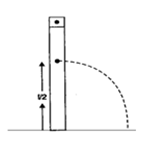{tex}\text{ }\!\!\omega\!\!\text{ =}\sqrt{\frac{\text{3gl}}{{{\text{l}}^{\text{2}}}}}\text{-}\sqrt{\frac{\text{3g}}{\text{l}}}{/tex}
v =lm
= {tex}\sqrt{3gl}{/tex}

9. State the laws of limiting friction.
Ans. The laws of limiting friction are as follows:
(a) The value of limiting friction depends on the nature of the two surfaces in contact and on the state of their smoothness.
(b) The force of friction acts tangential to the surfaces in contact in a direction opposite to the direction of relative motion.
(c) The value of limiting friction is directly proportional to the normal reaction between the two given surfaces.
(d) For any two given surface and for a given value of normal reaction the force of limiting friction is independent of the shape and surface area of surfaces in contact. Coefficient of limiting friction for two given surfaces in contact is defined as the ratio of the force of normal reaction N.
{tex}{{\mu }_{r}}=\frac{{{f}_{1}}}{N}{/tex}

10. If 1A0 = 10-10 m and the size of a hydrogen atom is about 0.5 A0, then what is the total atomic volume in m3 of a mole of hydrogen atoms?
Ans. Volume of the one hydrogen atom = {tex}\frac{4}{3}{/tex} πr3
={tex}\frac{4}{3}{/tex}  x 3.14 x (0.5 x 10-10) m3
=5.23 x 10-31m3
According to Avogadro’s hypothesis, one mole of hydrogen contains 6.023 x 1023 atoms.
Atomic volume of 1 mole of hydrogen atom = 6.023 x 1023 x 5.23 x 10-31
= 3.15 x 10-7m3.

11. What is the average speed during the whole journey, if a body covers half of its journey with a speed of 40 m/s and other half with a speed of 60 m/s.
Ans. Average speed = {tex}\frac{\text{Total Distance}}{\text{Timetaken}}{/tex}
Let x be the distance to be covered,
Average speed = {tex}\frac{x}{\frac{x}{2{{v}_{1}}}+\frac{x}{2{{v}_{2}}}}{/tex}
Where {tex}\frac{x}{2{{v}_{1}}}{/tex} = time taken to cover first half of the distance, {tex}\frac{x}{2{{v}_{2}}}{/tex} = time taken to cover the second half of the distance,
Average speed = {tex}\frac{x}{\frac{x\left( {{v}_{1}}+{{v}_{2}} \right)}{2{{v}_{1}}{{v}_{2}}}}{/tex}
= {tex}\frac{\text{x }\left( x \right)\text{ 2}{{\text{v}}_{\text{1}}}{{\text{v}}_{\text{2}}}}{\text{x}\left( {{\text{v}}_{\text{1}}}{{\text{v}}_{\text{2}}} \right)}\text{=}\frac{\text{2}{{\text{v}}_{\text{1}}}{{\text{v}}_{\text{2}}}}{{{\text{v}}_{\text{1}}}{{\text{v}}_{\text{2}}}}{/tex}
{tex}{{\text{V}}_{\text{av}}}\text{=}\frac{\text{2 x 40m/s x 60m/s}}{\text{100m/s}}{/tex}= 48 ms-1

12. Find the height to which it rises above the earth’s surface if a particle is projected vertically upwards from the surface of earth of radius R with kinetic energy equal to half of the minimum value needed for it to escape.
Ans. Escape velocity from the surface of earth is
{tex}{{\text{v}}_{\text{es}}}\text{=}\sqrt{\frac{\text{2GM}}{\text{R}}}{/tex}
K.E of a body Kes=1/2 mv2es = {tex}\frac{\text{GMm}}{\text{R}}{/tex}
The body is projected from the surface of earth with a K.E half of that needed to escape from earth surface hence
Initial K.E. of body {tex}\text{K = }\frac{{{\text{K}}_{\text{es}}}}{\text{2}}\text{ = }\frac{\text{GMm}}{\text{2R}}{/tex}
And its potential energy {tex}\text{ }\!\!\mu\!\!\text{ = – }\frac{\text{GMm}}{\text{R}}{/tex}
Total initial energy of body {tex}\text{-}\frac{\text{GMm}}{\text{2R}}{/tex}
The body goes up to a maximum height h from surface of earth, where the final K.E. =0 and P.E = {tex}\text{-}\frac{\text{GMm}}{\left( \text{R + h} \right)}{/tex}
Total energy = {tex}\text{-}\frac{\text{GMm}}{\left( \text{R + h} \right)}{/tex}
From conservation law of mechanical energy,
{tex}\text{-}\frac{\text{GMm}}{\text{2R}}{/tex} = {tex}\text{-}\frac{\text{GMm}}{\left( \text{R + h} \right)}{/tex}
On simplifying, h =R.

13. If the density of hydrogen at S.T.P is 0.09 kg m-3, then calculate
i. RMS velocity
ii. Mean kinetic energy E of one gram molecule of hydrogen at S.T.P.

Ans. Hence ρ = 0.09 kg m-3
S.T.P pressure P = 101 x 105 Pa.
According to K.E of gases
{tex}\text{P=}\frac{\text{1}}{\text{3}}\text{ }\!\!\rho\!\!\text{ }{{\text{C}}^{\text{2}}}\text{ or C=}\sqrt{\frac{\text{3P}}{\text{ }\!\!\rho\!\!\text{ }}}{/tex}
{tex}\sqrt{\frac{3×1.01x{{10}^{5}}}{0.09}}{/tex}
= 1837.5 ms-1
Volume occupied by one mole of hydrogen at S.T.P = 22.4 litres = 22.4 x 10-3m3
Mass of hydrogen M = volume x density
= 22.4 x 10-3 x 0.09
= 2.016 x 10-3 kg
Average K.E / mole = ½ MC2
= ½ x (2.016 x 10-3) x (1837.5)2
= 3403.4 J

14. The planet Mars has two moons, A and B
(i) How would you calculate the mass of Mars, A has a period 7 hours, 39 minutes and an orbital radius of 9.4 x 103 km
(ii) Assuming that Earth and Mars move in circular orbits around the sun with the Martian orbit being 1.52 times the orbital radius of the earth, then what is the length of the Martian year in days?

Ans. (i) The Sun’s Mass replaced by the Martian mass Mm
{tex}{{T}^{2}}=\frac{4{{\pi }^{2}}}{GMm}{{R}^{3}}{/tex}
{tex}Mm=\frac{4{{\pi }^{2}}}{G}\text{x}\frac{{{R}^{3}}}{{{T}^{2}}}{/tex}
{tex}Mm=\frac{4\text{x}{{(3.14)}^{2}}\text{x}{{(9.4)}^{3}}\text{x}{{10}^{18}}}{6.67\text{x}{{10}^{-11}}\text{x}{{(459\text{x}60)}^{2}}}{/tex}
{tex}=\frac{4\text{x}{{(3.14)}^{2}}\text{x}{{(9.4)}^{3}}\text{x}{{10}^{18}}}{6.67\text{x}{{(4.59\text{x}6)}^{2}}\text{x}{{10}^{-5}}}{/tex}
= 6.48 x 1023kg
(ii) Using Kepler’s third law
{tex}\frac{T_{M}^{2}}{T_{E}^{2}}=\frac{R_{MS}^{3}}{R_{ES}^{3}}{/tex}
Where RMS is the Mars (Earth) – Sun distance
{tex}{{T}_{M}}={{\left( \frac{{{R}_{MS}}}{{{R}_{ES}}} \right)}^{3/2}}-T_{E}^{2}{/tex}
= (1.52)3/2 x 365 = 684 days

15. Deduce the height at which the value of g is the same as at a depth of {tex}\frac{R}{2}{/tex}?
Ans. At depth =  {tex}\frac{R}{2}{/tex}, value of acceleration due to gravity
{tex}g’=g\left( 1-\frac{R}{2R} \right)=\frac{g}{2}{/tex}
At height x,
{tex}g’=g\left( 1-\frac{2x}{R} \right){/tex}
{tex}g\left( 1-\frac{2x}{R} \right)=\frac{g}{2}{/tex}
{tex}\frac{1}{2}=\frac{2x}{R}\Rightarrow x=\frac{R}{4}{/tex}

16. A body of mass m is suspended by two strings making angles α and β with the horizontal. Calculate the tensions in the two strings.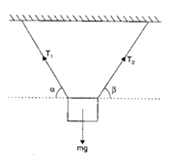Ans. Consider components of tensions T1 and T2 along the horizontal and vertical directions
– T1cos α + T2cos β = 0 ———————-    (i)
T1cos α = T2cos β ————————-     (ii)
T1 sin α + T2 sin β = mg
From (i)
{tex}{{\text{T}}_{\text{2}}}\text{=}\frac{{{\text{T}}_{\text{1}}}\text{cos }\!\!\alpha\!\!\text{ }}{\text{cos }\!\!\beta\!\!\text{ }}{/tex} And substituting if in (ii) we get
{tex}{{\text{T}}_{\text{1}}}\text{sin }\!\!\alpha\!\!\text{ +}\left( \frac{{{\text{T}}_{\text{1}}}\text{cos }\!\!\alpha\!\!\text{ }}{\text{cos }\!\!\beta\!\!\text{ }} \right)\text{sin }\!\!\beta\!\!\text{ = mg}{/tex}
{tex}{{\text{T}}_{\text{1}}}\frac{\text{sin}\left( \text{ }\!\!\alpha\!\!\text{ + }\!\!\beta\!\!\text{ } \right)}{\text{cos }\!\!\beta\!\!\text{ }}\text{ = mg}{/tex}
{tex}{{\text{T}}_{\text{1}}}\text{ = }\frac{\text{mgcos }\!\!\beta\!\!\text{ }}{\text{sin( }\!\!\alpha\!\!\text{ + }\!\!\beta\!\!\text{ )}}{/tex}
Hence, {tex}{{\text{T}}_{\text{2}}}\text{=}\frac{{{\text{T}}_{\text{1}}}\text{cos }\!\!\alpha\!\!\text{ }}{\text{cos }\!\!\beta\!\!\text{ }}\text{=}\frac{\text{mgcos }\!\!\beta\!\!\text{ }}{\text{sin( }\!\!\alpha\!\!\text{ + }\!\!\beta\!\!\text{ )}}\text{.}\frac{\text{cos }\!\!\alpha\!\!\text{ }}{\text{cos }\!\!\beta\!\!\text{ }}{/tex}
{tex}{{\text{T}}_{\text{2}}}\text{=}\frac{\text{mgcos }\!\!\alpha\!\!\text{ }}{\text{sin( }\!\!\alpha\!\!\text{ + }\!\!\beta\!\!\text{ )}}{/tex}

17. i. Calculate the angular momentum and rotational kinetic energy of earth about its own axis.
ii. How long could this amount of energy supply one KW power to each of the 3.5 x 109 persons on earth?

Ans. Assume that earth to be a solid sphere. We know that the moment of inertia of a solid sphere about its axis is
I = {tex}\frac{2}{5}{/tex}MR2
= {tex}\frac{2}{5}{/tex} x (6.0 x 1024kg) x (6.4 x 106m)2
= 9.8 x 1037 kg m2
In one day the earth completes one revolution. Hence the angular velocity is given by
{tex}\text{ }\!\!\omega\!\!\text{ = }\frac{\text{2 }\!\!\pi\!\!\text{ }}{\text{24 x 60 x 60}}\text{ = 7}\text{.27 x 1}{{\text{0}}^{\text{-5}}}\text{rad/sec}{/tex}
Angular momentum Iω = (98 x 1037 kg m2) (7.27 x 10-5 s-1)
= 7.1 x 1033 kg m2 / sec.
The rotational energy
{tex}\frac{1}{2}{/tex}Iω2 = {tex}\frac{1}{2}{/tex}(9.8 x 1037 kg m2) (7.27 x 10-5 s-1)2
= 2.6 x 1029 J
Power supplied by this energy
{tex}\text{P =}\frac{\text{Energy}}{\text{Time}}\text{ = }\frac{\text{2}\text{.6 x1}{{\text{0}}^{\text{29}}}}{\text{t}}\text{watt}{/tex}
{tex}\text{=}\frac{\text{2}\text{.6 x 1}{{\text{0}}^{\text{29}}}}{\text{1}{{\text{0}}^{\text{39}}}\text{t}}\text{KW}{/tex}
Power required by 3.5 x 109 persons = 3.5 x 109 x 1 kilowatt
{tex}\frac{\text{2}\text{.6 x 1}{{\text{0}}^{\text{29}}}}{\text{1}{{\text{0}}^{\text{3}}}\text{t}}\text{ = 3}\text{.5 x 1}{{\text{0}}^{\text{9}}}{/tex}
{tex}\text{t = }\frac{\text{2}\text{.6 x 1}{{\text{0}}^{\text{29}}}}{\text{1}{{\text{0}}^{\text{3}}}\text{ x 3}\text{.5 x 1}{{\text{0}}^{\text{9}}}}\text{sec}{/tex}
= 2.35 x 109 years

18. A diagram below is a light inextensible string. If a force F as shown acts upon M1, find the acceleration of the system and tension in string.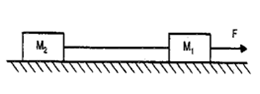Ans. As the string is inextensible acceleration of two blocks will be same. Also string is massless so tension throughout the string will be same. Contact force will be normal force only.
Acceleration of each block is a , tension in string is T and connect force between M1 and surface is N1 and contact force between M2 and surface is N2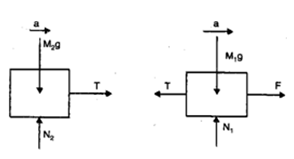Applying Newton’s second law for the blocks:
F – T = M1a————————–(i)
M1g – N1 =0————————–(ii)
T = M2a————————–(iii)
M2g – N2 =0————————–(iv)
Solving equation (i) and (iii)
{tex}\begin{align} & \text{a = }\frac{\text{F}}{{{\text{M}}_{\text{1}}}\text{+}{{\text{M}}_{\text{2}}}} \\ & \text{T = }\frac{{{\text{M}}_{\text{2}}}\text{F}}{{{\text{M}}_{\text{1}}}\text{+}{{\text{M}}_{\text{2}}}} \\ \end{align}{/tex}

19. i. State ideal gas equation.
ii. Draw graph to check whether a real gas obeys this equation.
iii. What is the conclusion drawn?

Ans. According to the ideal gas equation PV = µ RT
According to this equation
{tex}\frac{PV}{\mu T}{/tex} = R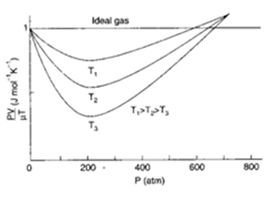Experimentally value of {tex}\frac{PV}{\mu T}{/tex} for real gas was calculated by altering the pressure of gas at different temperatures. The graphs obtained have been shown in the diagram. Here for the purpose of comparison, graph for an ideal gas has also been drawn, which is a straight line parallel to pressure axis.
From the graph it is clear that behavior of real gases is differ from an ideal gas. However at high temperatures and low pressures behaviors is nearly same as that of an ideal gas.

20. A uniform disc of radius R and mass M is mounted on an axis supported in fixed frictionless bearing. A light chord is wrapped around the rim of the wheel and supposes that we hang a body of mass m from the chord. Find the angular of the disc and tangential acceleration of point on the rim.
Ans. Let T be the tension in the chord
mg – T = ma,—————————-(i)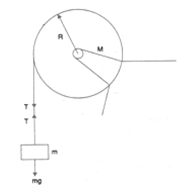Where a is the tangential acceleration of appoint on the rim of the disc.
τ =Iα
But the resultant torque on the disc = TR and the rotational inertia
I = ½ MR2
{tex}TR=\frac{1}{2}M{{R}^{2}}\left( \frac{a}{R} \right){/tex}
2TR = Ma
{tex}a=\frac{2T}{M}{/tex}————————— (ii)
From the equation (i) and (ii)
{tex}\text{mg -}\left( \frac{\text{Ma}}{\text{2}} \right)\text{ = ma}{/tex}
{tex}\text{a = }\left( \frac{\text{2m}}{\text{M + 2M}} \right)\text{g}{/tex}
{tex}\text{mg – T = m x}\left( \frac{\text{2T}}{\text{M}} \right){/tex}
{tex}\text{T = }\left( \frac{\text{mM}}{\text{M+2m}} \right)\text{g}{/tex}

21. The following equation represents standing wave set up in medium {tex}\text{y = 4 cos }\frac{\text{ }\!\!\pi\!\!\text{ x}}{\text{5}}\text{ sin 40 }\!\!\pi\!\!\text{ t}{/tex}, where x and y are in cm and t in seconds. Find out the amplitude and the velocity of the two component waves and calculate the distance between adjacent nodes. What is the velocity of a medium particle at x = 3cm at time {tex}\frac{1}{8}{/tex} second?
Ans. The equation of stationary wave is
{tex}\text{y = 4 cos }\frac{\text{ }\!\!\pi\!\!\text{ x}}{\text{3}}\text{ sin 40 }\!\!\pi\!\!\text{ t}{/tex}
{tex}\text{y = 2 x }2\cos \frac{2\pi \text{x}}{6}\sin \frac{2\text{x}(120)t}{6}{/tex}
We know that
{tex}y=2\text{a cos }\frac{\text{2 }\!\!\pi\!\!\text{ x}}{\text{ }\!\!\lambda\!\!\text{ }}\text{ sin }\frac{\text{2xvt}}{\text{ }\!\!\lambda\!\!\text{ }}{/tex}
By comparing tow equations we get,
a= 2cm, λ=6cm and v=220 cm/sec.
The component waves are
{tex}{{\text{y}}_{\text{1}}}\text{ = a sin}\frac{\text{2 }\!\!\pi\!\!\text{ }}{\text{ }\!\!\lambda\!\!\text{ }}\text{(vt-x)}{/tex}
{tex}{{\text{y}}_{\text{2}}}\text{ = a sin}\frac{\text{2 }\!\!\pi\!\!\text{ }}{\text{ }\!\!\lambda\!\!\text{ }}\text{(vt+x)}{/tex}
{tex}\text{= }\frac{\text{ }\!\!\lambda\!\!\text{ }}{\text{2}}\text{ = }\frac{\text{6}}{\text{2}}\text{ = 3cm}{/tex}
Particle velocity
{tex}\frac{\text{dy}}{\text{dt}}\text{ = 4 cos}\frac{\text{ }\!\!\pi\!\!\text{ x}}{\text{3}}\text{ cos (40 }\!\!\pi\!\!\text{ t)}\text{. 40 }\!\!\pi\!\!\text{ }{/tex}
= {tex}\text{160 cos }\frac{\text{ }\!\!\pi\!\!\text{ x}}{\text{3}}\text{cos 40 }\!\!\pi\!\!\text{ t}{/tex}
= {tex}\text{160 }\!\!\pi\!\!\text{ cos}\frac{\text{ }\!\!\pi\!\!\text{ x}}{\text{3}}\text{ cos}\left( \text{40 }\!\!\pi\!\!\text{ x }\frac{\text{1}}{\text{8}} \right){/tex}
=160 π
Particle velocity = 160 cm/sec.

22. Uniform spring whose upstretched length is {tex}l{/tex} has a force constant k. the spring is cut into two pieces of upstretched lengths, {tex}l{/tex} 1 and {tex}l{/tex} 2, where {tex}l{/tex} 1 = n {tex}l{/tex} 2 and n is an integer. What are the corresponding force constant K 1 and K 2 in terms of n and k?
Or
If a steel rod has a radius of 10 mm and a length of 1.0 m. A 100 kN force stretches it along its length, then calculate [Given Y = 2.0 x 1011N/m2] a) Stress
b) Elongation
c) Strain of the rod

Ans.
{tex}l{/tex} = {tex}l{/tex} 1+ {tex}l{/tex} 2—————————————– (i)
{tex}l{/tex} 1 = n {tex}l{/tex} 2—————————————- (ii)
{tex}\text{k =}\frac{\text{Mg}}{l}{/tex}————————————- (iii)
{tex}{{\text{k}}_{\text{1}}}\text{=}\frac{\text{Mg}}{{{l}_{\text{1}}}}{/tex}—————————— (iv)
{tex}{{\text{k}}_{\text{2}}}\text{=}\frac{\text{Mg}}{{{l}_{\text{2}}}}{/tex}——————————– (v)
Dividing the equation (iv) by (iii),
{tex}\frac{{{\text{k}}_{\text{1}}}}{\text{k}}=\frac{l}{{{l}_{2}}}=2\frac{{{l}_{1}}+{{l}_{2}}}{{{l}_{1}}}=1+\frac{{{l}_{2}}}{{{l}_{1}}}{/tex}
From the equation (ii) we find {tex}\frac{{{l}_{1}}}{{{l}_{2}}}{/tex} = n
{tex}\frac{{{\text{k}}_{\text{1}}}}{\text{k}}=1+\frac{1}{\text{n}}{/tex}
From equation (v) and (iii)
{tex}\frac{{{\text{k}}_{\text{2}}}}{\text{k}}=\frac{l}{{{l}_{2}}}=\frac{{{l}_{1}}+{{l}_{2}}}{{{l}_{2}}}=\frac{{{l}_{1}}}{{{l}_{2}}}+1{/tex}
From equation (ii) we have
{tex}\frac{{{l}_{1}}}{{{l}_{2}}}{/tex} = n
{tex}\frac{{{\text{k}}_{\text{2}}}}{\text{k}}\text{=}\left( \text{n+1} \right){/tex}
k2 = k(n+1)
Or
a) Stress = {tex}\frac{\text{F}}{\text{A}}\text{=}\frac{\text{F}}{\text{ }\!\!\pi\!\!\text{ }{{\text{r}}^{\text{2}}}}{/tex}
= 3.18 x 108 N/m2
b) Elongation {tex}\Delta {/tex}L = {tex}\frac{\left( \frac{\text{F}}{\text{A}} \right)\text{L}}{\text{Y}}{/tex}
= 1.59 mm
g) Strain = {tex}\frac{\text{ }\!\!\Delta\!\!\text{ L}}{\text{L}}{/tex}
= 0.16%

23. Radha was 70 kg at the age of 16 years. She then decided to lose weight. She started walking daily for 30 minutes and started taking only milk and fruits. Her parents advised her not to cut off her meal so drastically. She showed the result of losing 30 kg but she was feeling weak. So her parents took her to doctor who advised her to take proper balanced diet and exercise regularly.
a) Is taking crash diet advisable? Why?
b) Give the relation between SI and CGS unit of heat.
c) What would be her rise in temperature, if Radha weighing 40 kg now was advised to take 4000 kcal diet in a day and this energy was to be used in heating her without any losses? [Given: Sp. Heat of human body = 0.83 cal g-10C-1

Ans. a) Crash diet should not be taken as it makes body weak and less immune to diseases. It makes the body deficient in certain nutrients which is harmful for body.
b) The SI unit of heat = Joule and the CGS unit of heat = Calorie
1 calorie = 4.18 J
c) Substituting the values for {tex}\Delta {/tex}Q = cm{tex}\Delta {/tex}T, we get
{tex}\Delta {/tex}T = 40.160C

24. Find the expression for time period of motion of a body suspended by two springs connected in parallel and series.
Or
Calculate the frequency of the first and last fork if a set of 24 tuning forks is arranged in series of increasing frequencies. If each fork gives with the preceding one and the last fork is octave of the first.

Ans.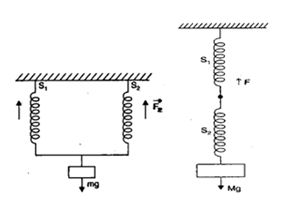Consider the body of mass M suspended by two springs connected in parallel. Let K 1 and K 2 be the spring constants of two springs.
Let the body be pulled down so that each spring is stretched through a distance y. Restoring   F 1 and F 2 will be developed in the springs S1 and S2.
According to Hooke’s law F 1 = – K 1y and F 2 = – K2y
Since both the forces acting in the same direction, total restoring force acting on the body is given by
F = F 1 + F 2 = – K 1 y – K 2 y = – (K 1 + K 2)y
Acceleration produced in the body is given by
{tex}\text{a = }\frac{\text{F}}{\text{M}}\text{ = – }\frac{\left( {{\text{K}}_{\text{1}}}\text{+}{{\text{K}}_{\text{2}}} \right)\text{Y}}{\text{M}}{/tex}————— (i)
Since {tex}\frac{\left( {{\text{K}}_{\text{1}}}\text{+ }{{\text{K}}_{\text{2}}} \right)}{\text{M}}{/tex} is constant a = – y
Hence motion of the body is SHM
The time period of body is given by
{tex}\text{T = 2 }\!\!\pi\!\!\text{ }\sqrt{\frac{\text{y}}{\left| \text{a} \right|}}\text{ = 2 }\!\!\pi\!\!\text{ }\sqrt{\frac{\text{M}}{{{\text{K}}_{\text{1}}}\text{+ }{{\text{K}}_{\text{2}}}}}{/tex}—————– (ii)
K 1 = K 2 = K
{tex}\text{T = 2 }\!\!\pi\!\!\text{ }\sqrt{\frac{\text{M}}{\text{2K}}}{/tex}
For series:
Consider the body of mass M suspended by two springs S 1 and S 2 which are connected in series. Let K 1 and K 2 be the spring constants of spring S1 and S2.
At any instant the displacement of the body from equilibrium position is y in the downward direction. If y 1 and y 2 be the extension produced in the springs S 1 and S 2.
y = y 1 + y 2 —————————-    (i)
Restoring the forces developed in S 1 and S 2 are given by,
F 1 = -K 1+ y 1 ———————————- (ii)
F 2 = -K 2+ y 2———————————- (iii)
Multiplying the equation (ii) by K 2 and equation (iii) by K 1 and adding we get,
K2F1+k1F2 = -k1k2(y 1+y 2) = -k1k2 y
Since both the springs are connected in series.
F 1 = F 2 =F
F (K 1+ K 2) =-k1k2y
{tex}\text{F = }\frac{{{\text{k}}_{\text{1}}}{{\text{k}}_{\text{2}}}}{\left( {{\text{k}}_{\text{1}}}\text{+ }{{\text{k}}_{\text{2}}} \right)\text{y}}{/tex}
If ‘a’ be the acceleration produced in the body of mass ‘M’ then,
{tex}\text{a = }\frac{\text{F}}{\text{M}}\text{ = }\frac{{{\text{k}}_{\text{1}}}{{\text{k}}_{\text{2}}}\text{y}}{\left( {{\text{k}}_{\text{1}}}\text{+ }{{\text{k}}_{\text{2}}} \right)\text{M}}{/tex}————————– (iv)
Time period of the body is given by,
{tex}\text{T = 2 }\!\!\pi\!\!\text{ }\sqrt{\frac{\text{y}}{\left| \text{a} \right|}}\text{ = 2 }\!\!\pi\!\!\text{ }\sqrt{\frac{\left( {{\text{k}}_{\text{1}}}\text{+}{{\text{k}}_{\text{2}}} \right)\text{M}}{{{\text{k}}_{\text{1}}}{{\text{k}}_{\text{2}}}}}{/tex}
{tex}\text{T = 2 }\!\!\pi\!\!\text{ }\sqrt{\left( \frac{\text{1}}{{{\text{k}}_{\text{1}}}}\text{+}\frac{\text{1}}{{{\text{k}}_{\text{2}}}} \right)}\text{M}{/tex}
Or
Let the frequency of the first tuning fork be n. as last fork is octave of the first therefore frequency of the last fork = 2n
As each fork gives 4 beats / seconds with the preceding one,
Therefore, frequency of 2nd fork = n + 4
Frequency of the 3rd fork = n +8 = n +4(2) = n + 4(3-1)
Proceeding in this way, frequency of 24th fork = n + 4(24 -1) = n +92
2n = n + 92 or n = 92 Hz.
Frequency of the last fork = 2n = 2 x 92 = 184 Hz.

25. Explain the kinematic equation for uniformly accelerated motion.
Or
A particle is thrown over a triangle from one end of a horizontal base that grazing h vertex fall on the other end of the base. If α and β be the same angles and {tex}\theta {/tex} the angle of projection, prove that tan {tex}\theta {/tex} = tan α + tan β.

Ans. Uniformly accelerated motion, we can drive some simple equation that relate displacements (x), time taken (f), initial velocity (u), final velocity (v) and acceleration (a).
(i) Velocity attends after time t: the velocity-time graph for positive constant acceleration of a particle.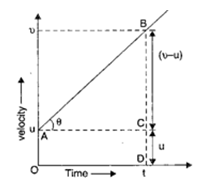Let u be the initial velocity of the particle at l =0 and v is the final velocity of the particle after time t. consider two points A and B on the curve corresponding to t=0 and t =t.
Draw ZBD perpendicular to time axis. Also draw AC perpendicular to BD.
OA = CD = u
BC = (v – u) and OD = t
Now,
Slope of v – t graph = acceleration (a)
a = slope of v – t graph
tan{tex}\theta {/tex} = {tex}\frac{BC}{AC}=\frac{BC}{OD}{/tex}
{tex}\text{a = }\frac{\text{v – u}}{\text{t}}{/tex}
v –u = at
v = u + at
(ii)    Distance travelled in time t will be,
x0 = position of the particle at t =0 from the origin
x = position of the particle at t =t from the origin
(x – x0) = S = distance travelled by the particle in the time interval (t -0) =t
Distance travelled by a particle in the given time
Interval = area under velocity-time graph
(x – x0) = area OABD
= area of Trapezium OABD
= ½ [Sum of parallel sides x perpendicular distance between parallel sides] = ½ (OA + BZD) x AC
= ½ (u + v) x t
v =u +at
(x –x0) = ½ (u + u + at) x t
= ½ (2u + at) x t
= ut + ½ at2
x – x0 = S
S = ut + ½ at2
(iii)    Velocity attained after travelling a distance S:
Distance travelled by a particle in time t is equal to the area under velocity-time graph. The distance (s) travelled by a particle during time interval t is given by
S = area under v – t graph
S = area of Trapezium OABD
= ½ (sum of parallel sides) x perpendicular distance between these parallel sides
S = ½ (OA + OD) x AC    ————————— (i)
Acceleration a = slope of v – t graph
{tex}A=\frac{BC}{AC}=\frac{BD-CD}{AC}=\frac{v-u}{AC}{/tex}
{tex}\text{AC = }\left( \frac{\text{v – u}}{\text{a}} \right){/tex}—————————- (ii)
OA = u and BD = v————————— (iii)
From equation (i), (ii) and (iii) we get
{tex}\text{S = }\frac{\text{1}}{\text{2}}\left( \text{v+u} \right)\frac{\left( \text{v-u} \right)}{\text{a}}{/tex}
{tex}\text{S = }\frac{{{\text{v}}^{\text{2}}}\text{- }{{\text{u}}^{\text{2}}}}{\text{2a}}{/tex}
v2 – u2 = 2aS
Or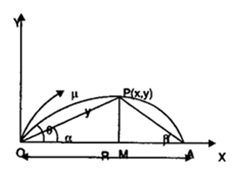From the
{tex}\begin{align} & \text{tan }\!\!\alpha\!\!\text{ = }\frac{\text{y}}{\text{x}} \\ & \text{tan }\!\!\beta\!\!\text{ = }\frac{\text{y}}{\text{MA}}\text{ = }\frac{\text{y}}{\text{R – x}} \\ \end{align}{/tex}
where R is horizontal range.
{tex}\text{tan }\!\!\alpha\!\!\text{ + tan }\!\!\beta\!\!\text{ = }\frac{\text{y}}{\text{x}}\text{+}\frac{\text{y}}{\text{R – x}}{/tex}
= {tex}\frac{\left( \text{R – x + x} \right)\text{y}}{\text{x}\left( \text{R – x} \right)}\text{=}\frac{\text{yR}}{\text{x}\left( \text{R – x} \right)}{/tex}
{tex}\text{tan }\!\!\alpha\!\!\text{ + tan }\!\!\beta\!\!\text{ = }\frac{\text{yR}}{\text{x}\left( \text{R – x} \right)}{/tex}——————————-     (i)
{tex}\text{x = }\left( \text{u cos }\!\!\theta\!\!\text{ } \right)\text{t}{/tex}——————————-    (ii)
{tex}\text{y = }\left( \text{u sin }\!\!\theta\!\!\text{ } \right)\text{t – }\frac{\text{1}}{\text{2}}\text{g}{{\text{t}}^{\text{2}}}{/tex}——————–     (iii)
From equation (ii) and (iii),
{tex}\text{y = x tan }\!\!\theta\!\!\text{ }\left[ \text{1- }\frac{\text{xg}}{\text{2}{{\text{u}}^{\text{2}}}\text{co}{{\text{s}}^{\text{2}}}\text{ }\!\!\theta\!\!\text{ tan }\!\!\theta\!\!\text{ }} \right]{/tex}
Substituting,
{tex}\text{R = }\frac{\text{2}{{\text{u}}^{\text{2}}}\text{sin }\!\!\theta\!\!\text{ cos }\!\!\theta\!\!\text{ }}{\text{g}}{/tex}
{tex}\text{y = x tan }\!\!\theta\!\!\text{ }\left[ \text{1 – }\frac{\text{xg}}{\text{2}{{\text{u}}^{\text{2}}}\text{cos } \!\!\theta\!\!\text{ sin }\!\!\theta\!\!\text{ }} \right]{/tex}
{tex}\text{y = x tan }\!\!\theta\!\!\text{ }\left[ \text{1-}\frac{\text{x}}{\text{R}} \right]{/tex}
{tex}\frac{\text{y}}{\text{x}}\text{= tan }\!\!\theta\!\!\text{ }\left( \frac{\text{R – x}}{\text{R}} \right){/tex}——————— (iv)
Putting (iv) in (i) we get,
{tex}\text{tan }\!\!\alpha\!\!\text{ + tan }\!\!\beta\!\!\text{ = }\frac{\text{yR}}{\text{x}\left( \text{R – X} \right)}\text{ = tan }\!\!\theta\!\!\text{ }{/tex}
{tex}\tan \alpha +\tan \beta =\tan \theta {/tex}

26. If a stone is dropped from the top of a mountain and n second later another stone is thrown vertically downwards with a velocity of u m/s, then how far below the top of the mountain will be the second stone overtake the first?
Or
A particle is projected horizontally with a speed u from top of a plane inclined at an angle {tex}\theta {/tex} with the horizontal direction. How far from the point of projection will the particle strike the plane?

Ans. The second stone will catch up with the first stone when the distance covered by it in (t – n) second will equal the distance covered by the first stone in t second.
The distance covered by the first stone in t second = ½ gt2 and distance covered by the second stone in (1 –n) second.
u (t –n) + ½ g (t –n)2
½ gt2 = u (t – n) + ½ g (t –n)2
½ g [t2 – (t-n)2] = u (t –n)
½ g [(2t –n) n] = u (t –n)
gnt – ½ gn2 = ut –un
t (gn – u) = ( ½ gn –u)n
{tex}\text{t = }\frac{\text{n}\left( \frac{\text{1}}{\text{2}}\text{gn – u} \right)}{\left( \text{gn – u} \right)}{/tex}
The distance covered by the first stone in this time
{tex}\text{h =}\frac{\text{1}}{\text{2}}\text{g}{{\text{t}}^{\text{2}}}\text{ = }\frac{\text{1}}{\text{2}}\text{g}\left[ \frac{\text{n}\left( \frac{\text{1}}{\text{2}}\text{gn – u} \right)}{\left( \text{gn – u} \right)} \right]{/tex}
Thus the second stone will overtake the first at distance
{tex}\frac{\text{1}}{\text{2}}\text{g}{{\left[ \frac{\text{n}\left( \frac{\text{gn}}{\text{2}}\text{- u} \right)}{\left( \text{gn – u} \right)} \right]}^{\text{2}}}{/tex}
Or
Let the particle projected from O strike the inclined plane OA at P after time t and coordinates of P be (x,y).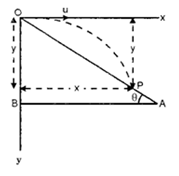Taking motion of projectile from O to P along x-axis we have
x0 = 0, x = x, ux = u, ax =0, t=t
Using the relation x = x0 + uxt + ½ axt2
We get x = ut or i =x/u
Taking motion of projectile along y – axis
y0 = y, y = y, u = 0, ay = g, t = t
Using the relation y = y0 + uyt + ½ ayt2
{tex}\text{y = 0 + 0 + }\frac{\text{1}}{\text{2}}\text{ g}{{\text{t}}^{\text{2}}}\text{ = }\frac{\text{1}}{\text{2}}\text{g}{{\text{t}}^{\text{2}}}\text{ = }\frac{\text{1}}{\text{2}}\text{g}\frac{{{\text{x}}^{\text{2}}}}{{{\text{u}}^{\text{2}}}}{/tex}
y = x tan {tex}\theta {/tex}, so gx2 / 2u2 = x tan{tex}\theta {/tex}
x = {tex}\frac{\text{2}{{\text{u}}^{\text{2}}}\text{tan }\!\!\theta\!\!\text{ }}{\text{g}}{/tex}
And y = x tan{tex}\theta {/tex} = {tex}\frac{\text{2}{{\text{u}}^{\text{2}}}\text{ta}{{\text{n}}^{\text{2}}}\text{ }\!\!\theta\!\!\text{ }}{\text{g}}{/tex}
Distance OP = {tex}\sqrt{{{\text{x}}^{\text{2}}}\text{+ }{{\text{y}}^{\text{2}}}}{/tex}
= {tex}\frac{2{{u}^{2}}\tan \theta }{g}\sqrt{1+{{\tan }^{2}}\theta }{/tex}
= {tex}\frac{\text{2}{{\text{u}}^{\text{2}}}\text{ tan }\!\!\theta\!\!\text{ sec }\!\!\theta\!\!\text{ }}{\text{g}}{/tex}

This is only initial part of the whole sample paper. Download Complete set of sample paper for class 11 Physics

## Sample Paper for class 11

It is Sample paper for class 11 Physics. However, myCBSEguide provides the best sample papers for all the subjects. There are number of sample papers which you can download from myCBSEguide website. Sample paper for class 11 all subjects

These are also Sample Paper for class 11 Physics available for download through myCBSEguide app. These are the latest Sample Paper for class 11 Physics as per the new exam pattern. Download the app today to get the latest and up-to-date study material.

## Marking Scheme for Class 11 exam

 Subject Board Marks Practical or internal Marks English 100 Marks ZERO Marks Hindi 100 Marks ZERO Marks Mathematics 100 Marks ZERO Marks Chemistry 70 Marks 30 Marks Physics 70 Marks 30 Marks Biology 70 Marks 30 Marks Computer Science 70 Marks 30 Marks Informatics Practices 70 Marks 30 Marks Accountancy 80 Marks 20 Marks Business Studies 80 Marks 30 Marks Economics 80 Marks 20 Marks History 80 Marks 20 Marks Political Science 100 Marks ZERO Marks Geography 70 Marks 30 Marks Sociology 80 Marks 20 Marks Physical Education 70 Marks 30 Marks Home Science 70 Marks 30 Marks

To download complete sample paper for class 11 Physics, Business Studies, Political Science, Economics, Biology, Geography, Computer Science, Home Science, Maths and Chemistry; do check myCBSEguide app or website. myCBSEguide provides sample papers with solution, test papers for chapter-wise practice, NCERT solutions, NCERT Exemplar solutions, quick revision notes for ready reference, CBSE guess papers and CBSE important question papers. Sample Paper all are made available through the best app for CBSE students and myCBSEguide website.### Test Generator

Create question paper PDF and online tests with your own name & logo in minutes.### myCBSEguide

Question Bank, Mock Tests, Exam Papers, NCERT Solutions, Sample Papers, Notes